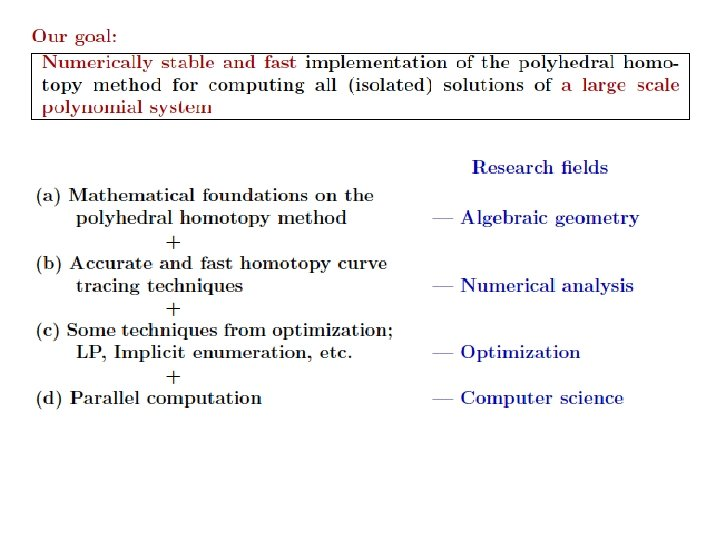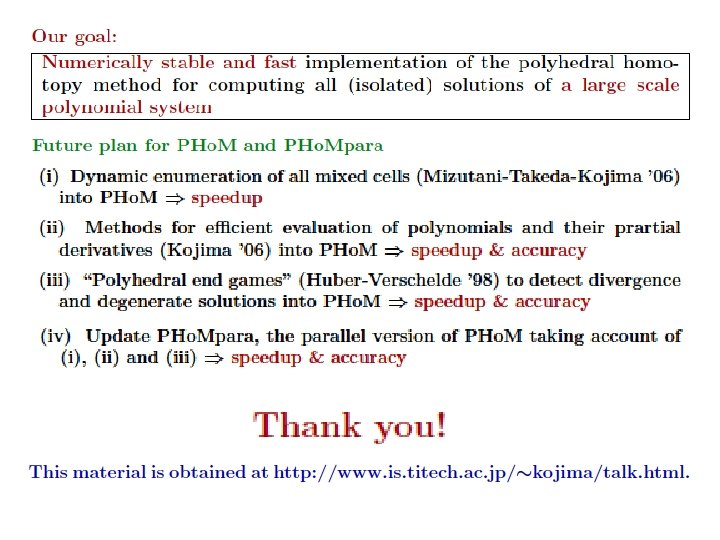# Parallel Implementation of the Polyhedral Homotopy Method IMA

• Slides: 36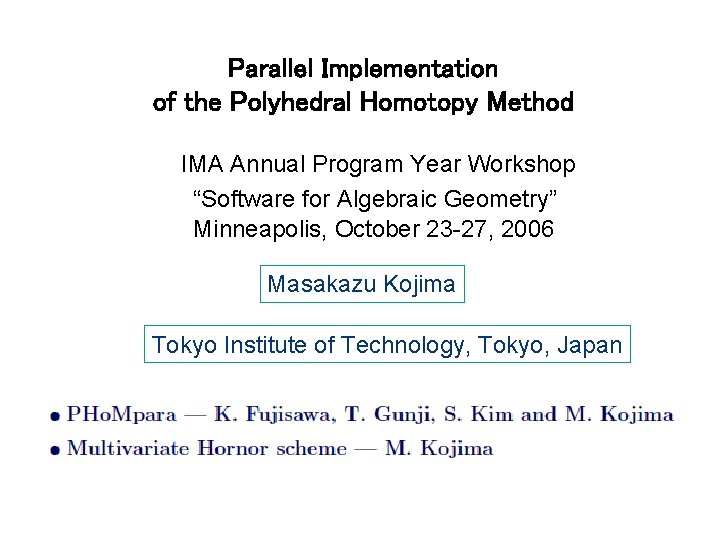Parallel Implementation of the Polyhedral Homotopy Method IMA Annual Program Year Workshop “Software for Algebraic Geometry” Minneapolis, October 23 -27, 2006 Masakazu Kojima Tokyo Institute of Technology, Tokyo, Japan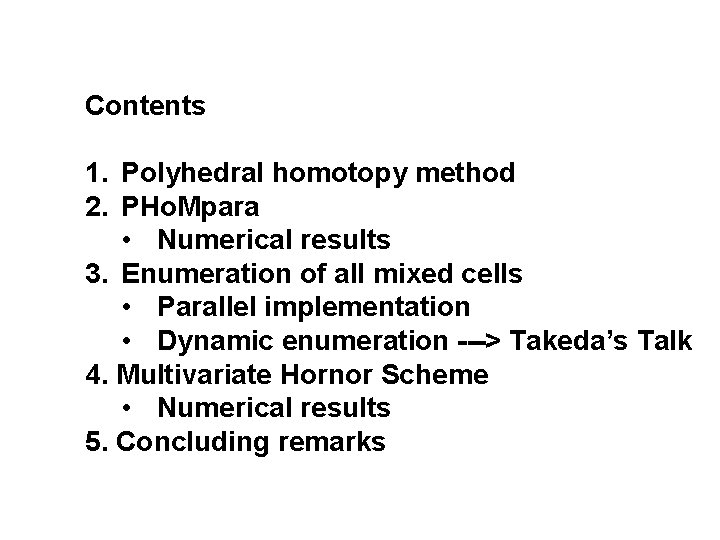Contents 1. Polyhedral homotopy method 2. PHo. Mpara • Numerical results 3. Enumeration of all mixed cells • Parallel implementation • Dynamic enumeration ---> Takeda’s Talk 4. Multivariate Hornor Scheme • Numerical results 5. Concluding remarksContents 1. Polyhedral homotopy method 2. PHo. Mpara • Numerical results 3. Enumeration of all mixed cells • Parallel implementation • Dynamic enumeration ---> Takeda’s Talk 4. Multivariate Hornor Scheme • Numerical results 5. Concluding remarks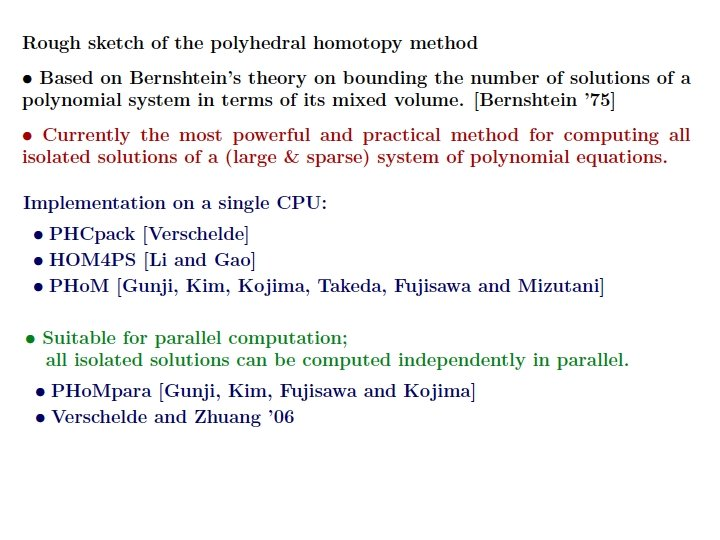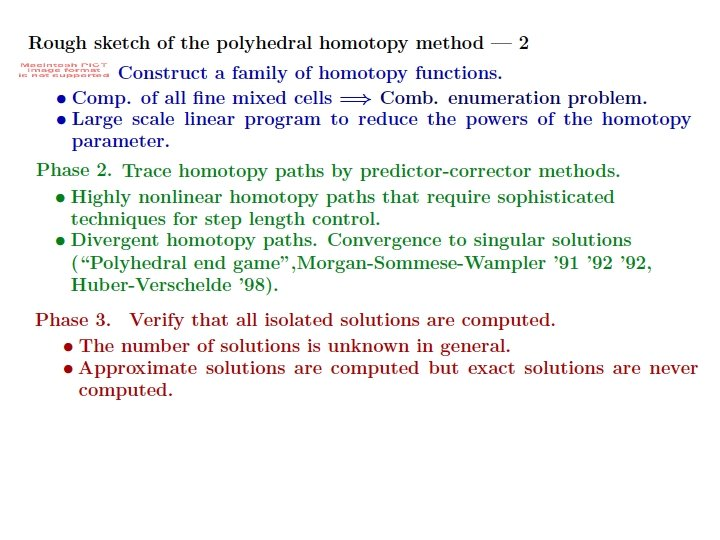Contents 1. Polyhedral homotopy method 2. PHo. Mpara • Numerical results 3. Enumeration of all mixed cells • Parallel implementation • Dynamic enumeration ---> Takeda’s Talk 4. Multivariate Hornor Scheme • Numerical results 5. Concluding remarks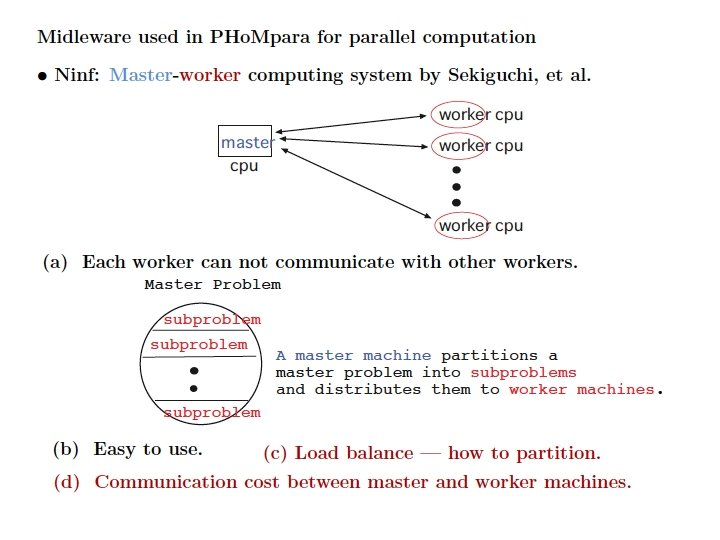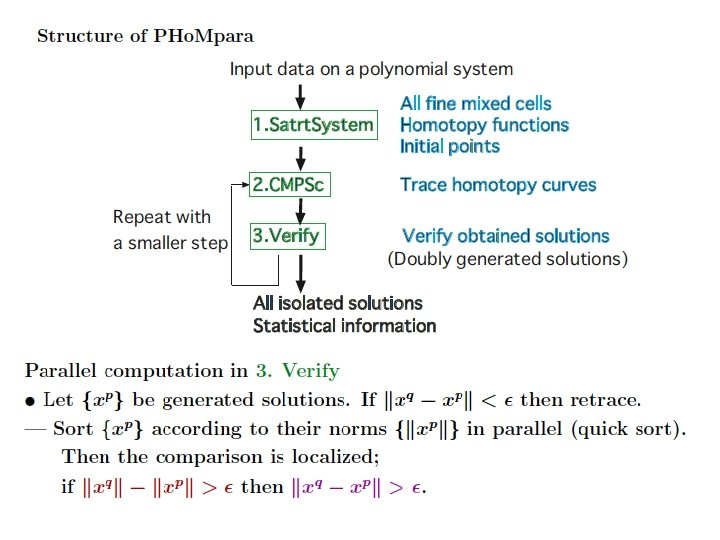Contents 1. Polyhedral homotopy method 2. PHo. Mpara • Numerical results 3. Enumeration of all mixed cells • Parallel implementation • Dynamic enumeration ---> Takeda’s Talk 4. Multivariate Hornor Scheme • Numerical results 5. Concluding remarks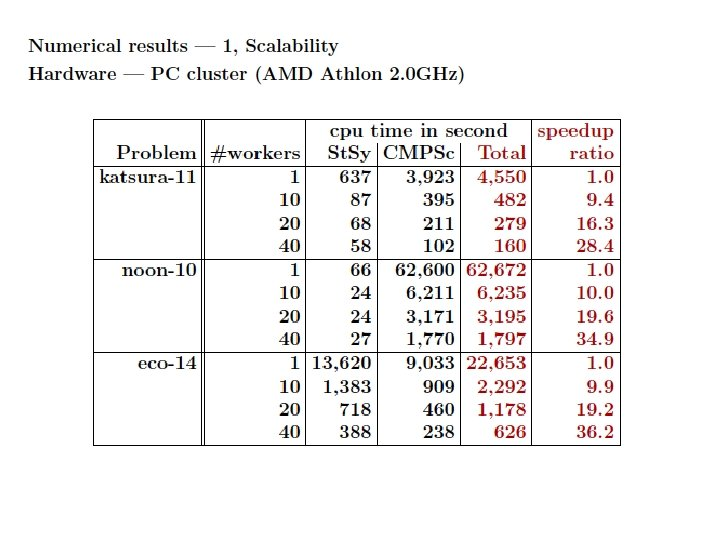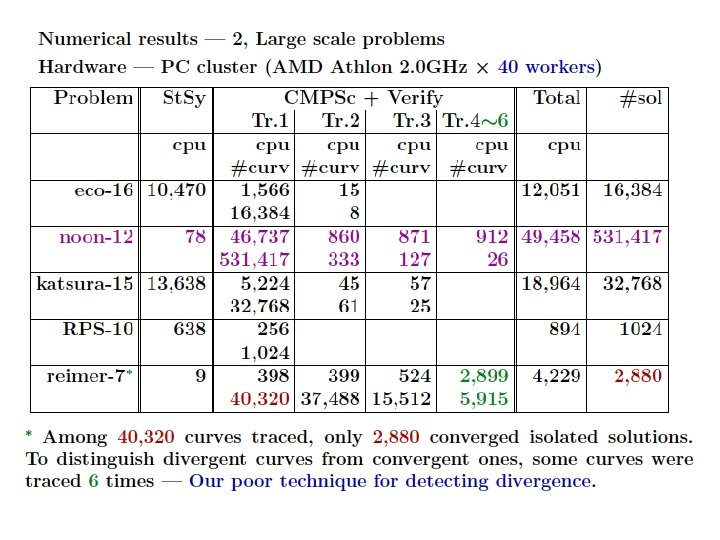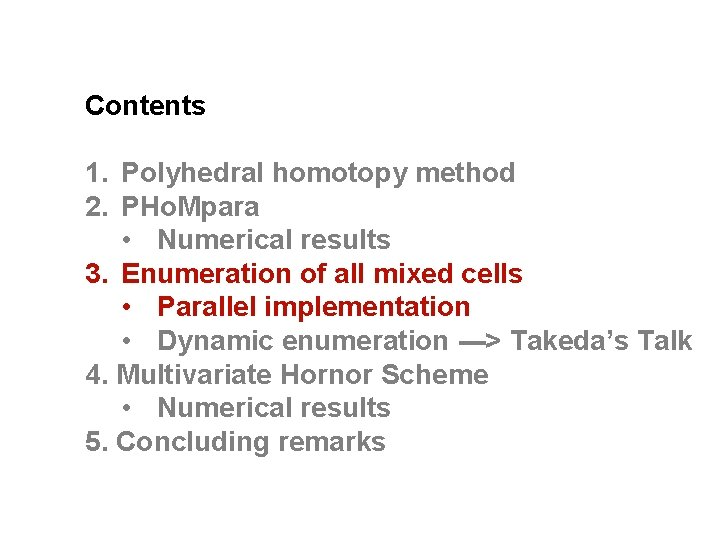Contents 1. Polyhedral homotopy method 2. PHo. Mpara • Numerical results 3. Enumeration of all mixed cells • Parallel implementation • Dynamic enumeration ---> Takeda’s Talk 4. Multivariate Hornor Scheme • Numerical results 5. Concluding remarks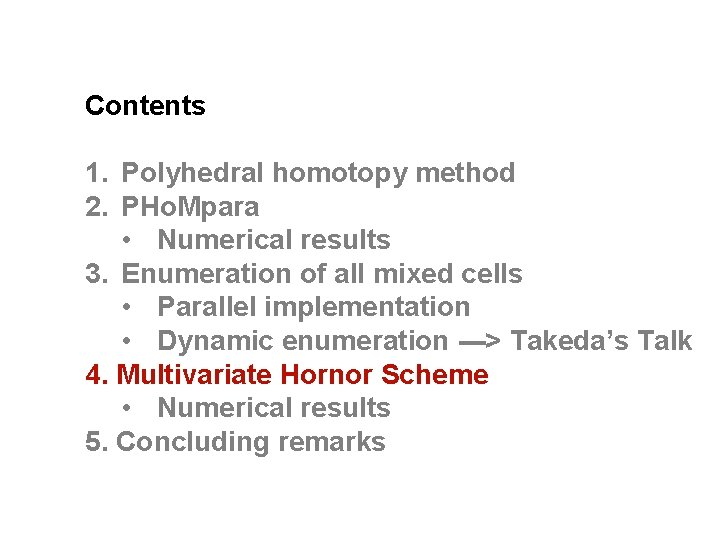Contents 1. Polyhedral homotopy method 2. PHo. Mpara • Numerical results 3. Enumeration of all mixed cells • Parallel implementation • Dynamic enumeration ---> Takeda’s Talk 4. Multivariate Hornor Scheme • Numerical results 5. Concluding remarks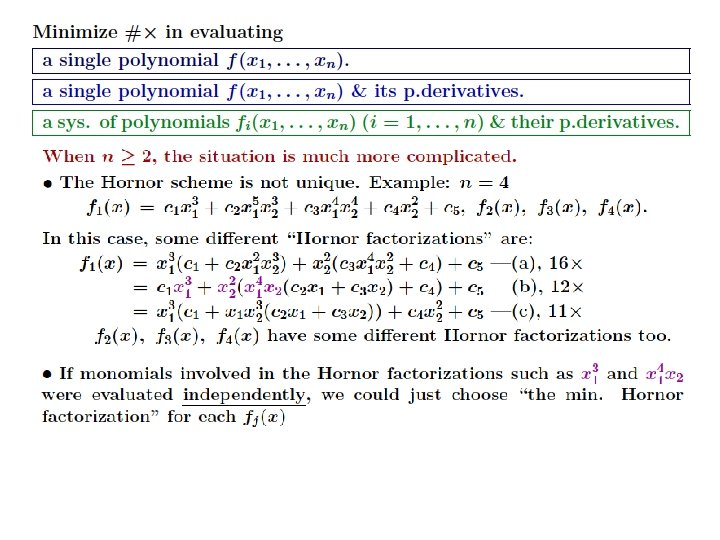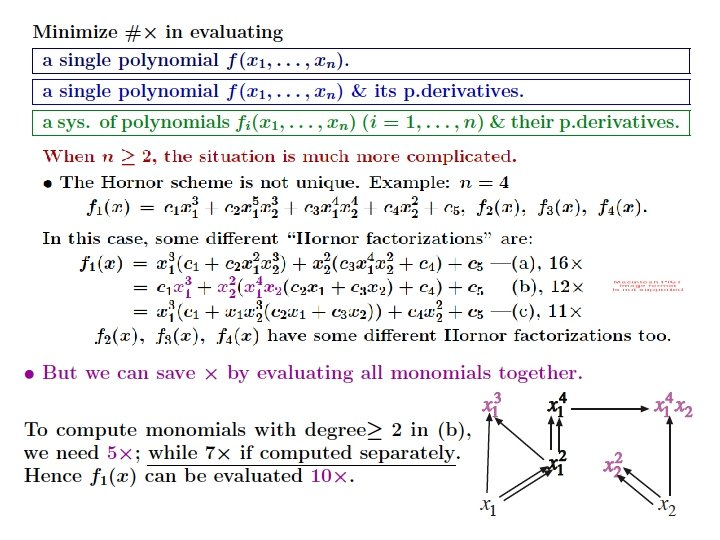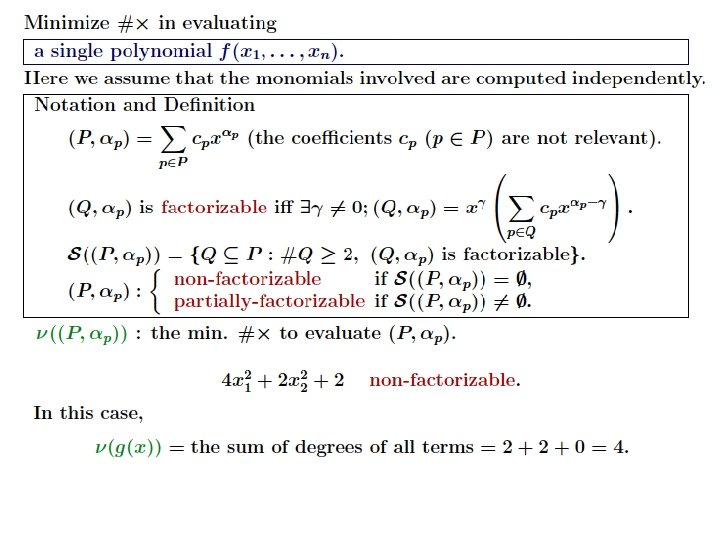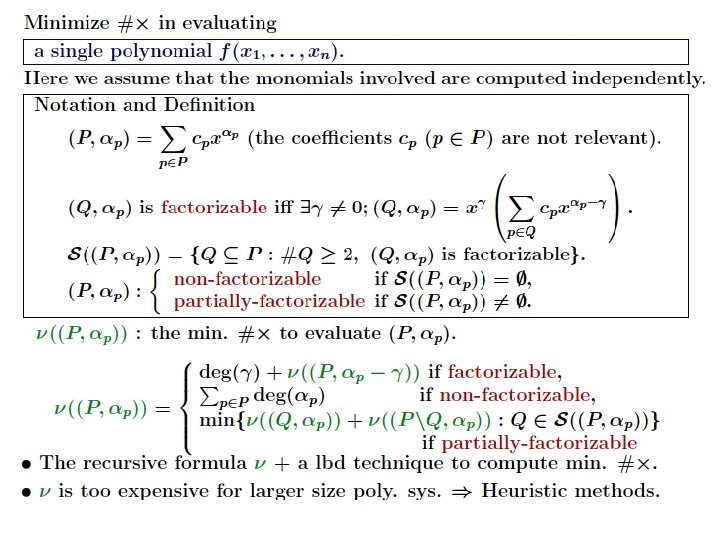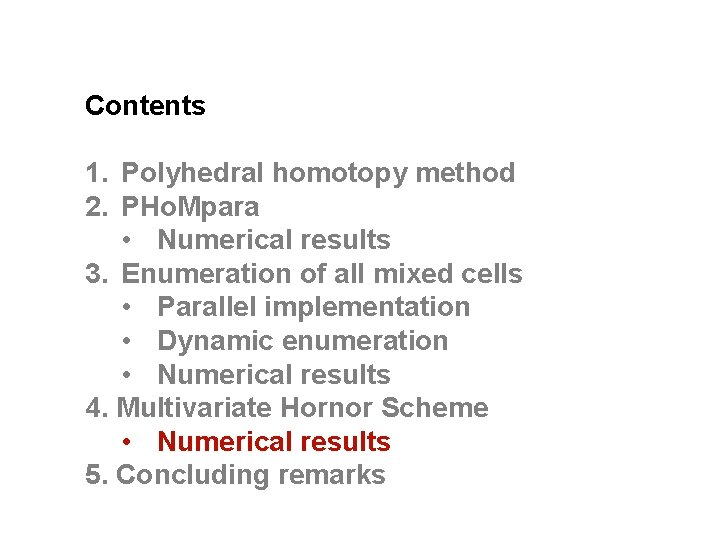Contents 1. Polyhedral homotopy method 2. PHo. Mpara • Numerical results 3. Enumeration of all mixed cells • Parallel implementation • Dynamic enumeration • Numerical results 4. Multivariate Hornor Scheme • Numerical results 5. Concluding remarks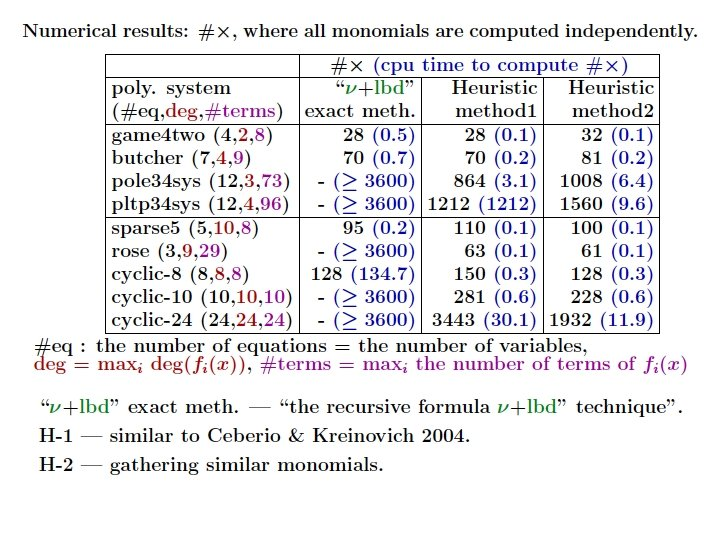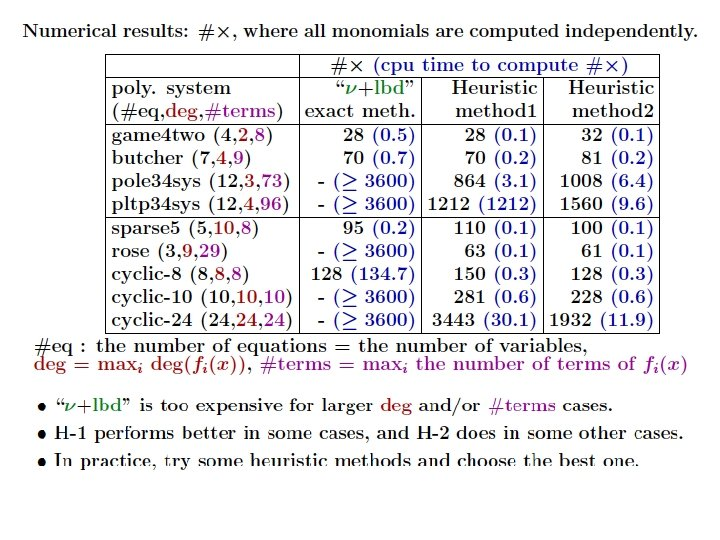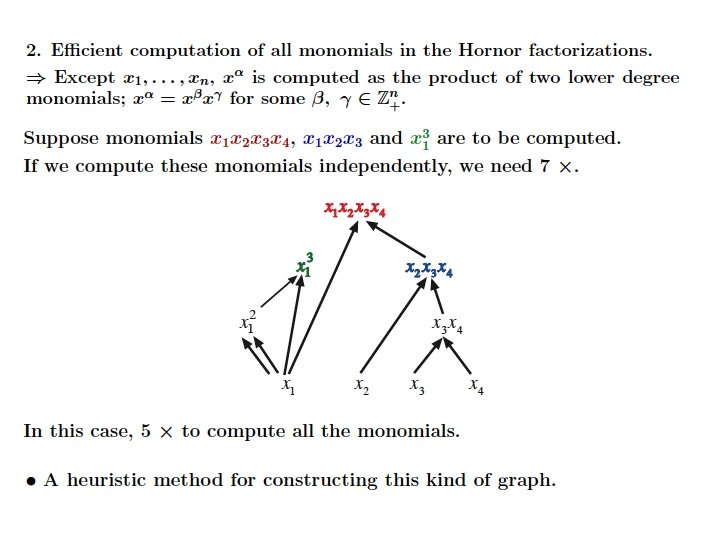Contents 1. Polyhedral homotopy method 2. PHo. Mpara • Numerical results 3. Enumeration of all mixed cells • Parallel implementation • Dynamic enumeration ---> Takeda’s Talk 4. Multivariate Hornor Scheme • Numerical results 5. Concluding remarks